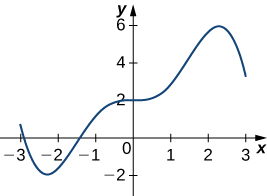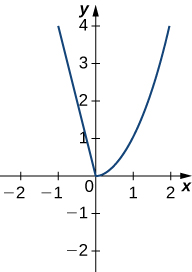## Chapter 3 Review Exercises

True or False? Justify the answer with a proof or a counterexample.

1. Every function has a derivative.

2. A continuous function has a continuous derivative.

3. A continuous function has a derivative.

4. If a function is differentiable, it is continuous.

Use the limit definition of the derivative to exactly evaluate the derivative.

5. $f(x)=\sqrt{x+4}$

6. $f(x)=\frac{3}{x}$

Find the derivatives of the following functions.

7. $f(x)=3x^3-\frac{4}{x^2}$

8. $f(x)=(4-x^2)^3$

9. $f(x)=e^{\sin x}$

10. $f(x)=\ln(x+2)$

11. $f(x)=x^2 \cos x+x \tan x$

12. $f(x)=\sqrt{3x^2+2}$

13. $f(x)=\frac{x}{4} \sin^{-1} x$

14. $x^2 y=(y+2)+xy \sin (x)$

Find the following derivatives of various orders.

15. First derivative of $y=x \ln x \cos x$

16. Third derivative of $y=(3x+2)^2$

17. Second derivative of $y=4^x+x^2 \sin x$

Find the equation of the tangent line to the following equations at the specified point.

18. $y= \cos^{-1} x+x$ at $x=0$

19. $y=x+e^x-\frac{1}{x}$ at $x=1$

Draw the derivative for the following graphs.

20.21.The following questions concern the water level in Ocean City, New Jersey, in January, which can be approximated by $w(t)=1.9+2.9 \cos (\frac{\pi}{6}t)$, where $t$ is measured in hours after midnight, and the height is measured in feet.

22. Find and graph the derivative. What is the physical meaning?

23. Find $w^{\prime}(3)$. What is the physical meaning of this value?

The following questions consider the wind speeds of Hurricane Katrina, which affected New Orleans, Louisiana, in August 2005. The data are displayed in a table.

Wind Speeds of Hurricane Katrina
Source: http://news.nationalgeographic.com/news/2005/09/0914_050914_katrina_timeline.html.
Hours after Midnight, August 26 Wind Speed (mph)
1 45
5 75
11 100
29 115
49 145
58 175
73 155
81 125
85 95
107 35

24. Using the table, estimate the derivative of the wind speed at hour 39. What is the physical meaning?

25. Estimate the derivative of the wind speed at hour 83. What is the physical meaning?